# Converting Bases | Conversion of Bases

## Number System Conversions-

Before you go through this article, make sure that you have gone through the previous article on Basics of Number System.

In number system,

• It is very important to have a good knowledge of how to convert numbers from one base to another base.
• Here, we will learn how to convert any given number from any base to any other base.## Conversion of Bases-

A given number in base x can be converted to any other base y using the following steps-

### Step-01:

Convert the number from base x to base 10 using expansion method.

Read More- Conversion to Base 10

### Step-02:

Convert the number from base 10 to base y using division & multiplication method.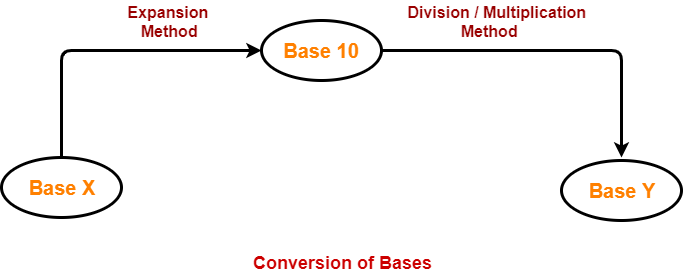## Problem-01:

Convert (1056)16 to ( ? )8

## Solution-

### (1056)16 → ( ? )10

Using Expansion method, we have-

(1056)16

= 1 x 163 + 0 x 162 + 5 x 161 + 6 x 160

= 4096 + 0 + 80 + 6

= (4182)10

From here, (1056)16 = (4182)10

### (4182)10 → ( ? )8

Using Division method, we have-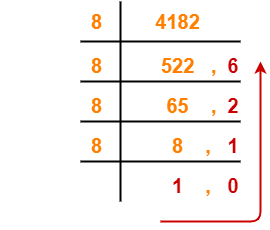From here, (4182)10 = (10126)8

Thus, (1056)16 = (10126)8

## Problem-02:

Convert (11672)8 to ( ? )16

## Solution-

### (11672)8 → ( ? )10

Using Expansion method, we have-

(11672)8

= 1 x 84 + 1 x 83 + 6 x 82 + 7 x 81 + 2 x 80

= 4096 + 512 + 384 + 56 + 2

= (5050)10

From here, (11672)8 = (5050)10

### (5050)10 → ( ? )16

Using Division method, we have-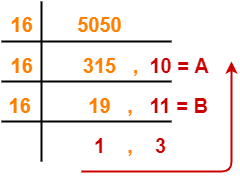From here, (5050)10 = (13BA)16

Thus, (11672)8 = (13BA)16

## Problem-03:

Convert (2724)8 to ( ? )5

## Solution-

### (2724)8 → ( ? )10

Using Expansion method, we have-

(2724)8

= 2 x 83 + 7 x 82 + 2 x 81 + 4 x 80

= 1024 + 448 + 16 + 4

= (1492)10

From here, (2724)8 = (1492)10

### (1492)10 → ( ? )5

Using Division method, we have-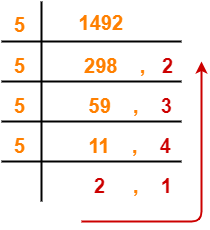From here, (1492)10 = (21432)5

Thus, (2724)8 = (21432)5

## Problem-04:

Convert (3211)4 to ( ? )5

## Solution-

### (3211)4 → ( ? )10

Using Expansion method, we have-

(3211)4

= 3 x 43 + 2 x 42 + 1 x 41 + 1 x 40

= 192 + 32 + 4 + 1

= (229)10

From here, (3211)4 = (229)10

### (229)10 → ( ? )5

Using Division method, we have-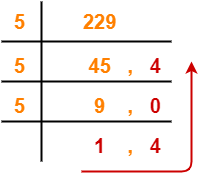From here, (229)10 = (1404)5

Thus, (3211)4 = (1404)5

## Problem-05:

Convert (1001001100)2 to ( ? )6

## Solution-

### (1001001100)2 → ( ? )10

Using Expansion method, we have-

(1001001100)2

= 1 x 29 + 0 x 28 + 0 x 27 + 1 x 26 + 0 x 25 + 0 x 24 + 1 x 23 + 1 x 22 + 0 x 21 + 0 x 20

= 512 + 64 + 8 + 4

= (588)10

From here, (1001001100)2 = (588)10

### (588)10 → ( ? )6

Using Division method, we have-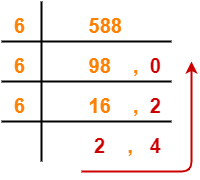From here, (588)10 = (2420)6

Thus, (1001001100)2 = (2420)6

To gain better understanding about Conversion of Bases,

Watch this Video Lecture

Get more notes and other study material of Number System.

Watch video lectures by visiting our YouTube channel LearnVidFun.

SummaryArticle Name
Converting Bases | Conversion of Bases
Description
Conversion of Bases- To convert a number from any base to any other base, it is first converted to base 10 using expansion method and then it is converted from base 10 to required base using division & multiplication method.
Author
Publisher Name
Gate Vidyalay
Publisher Logo